# Bayesian Glossary

When reading Bayesian texts, or listening to lectures, terms like "posterior" or "data" are used, but often without explanation leaving the audience confused. I also find that even after learning the concepts once, its easy to mix them up. To that end I put together this glossary to serve as a quick reference to the terms, with examples. The writing is colloquial, for precise definitions I recommend the following referencess

Terms are listed in order of relative importance. Use your browser's find feature if you're looking for a specific one.

## Motivating Example: Proportion of water on a globe¶

We will be using this example from Richard McElreath to help define all terms. In his example, we want to know the proportion of water on a given globe. We make estimations by tossing a globe, catching it, and seeing whether our right index finger is on water (W) or Land (L)

For further background I highly suggest purchasing his book and watching his lectures. Professor McElreath is an excellent author and lecturer

Recorded Lecture: https://youtu.be/XoVtOAN0htU?t=331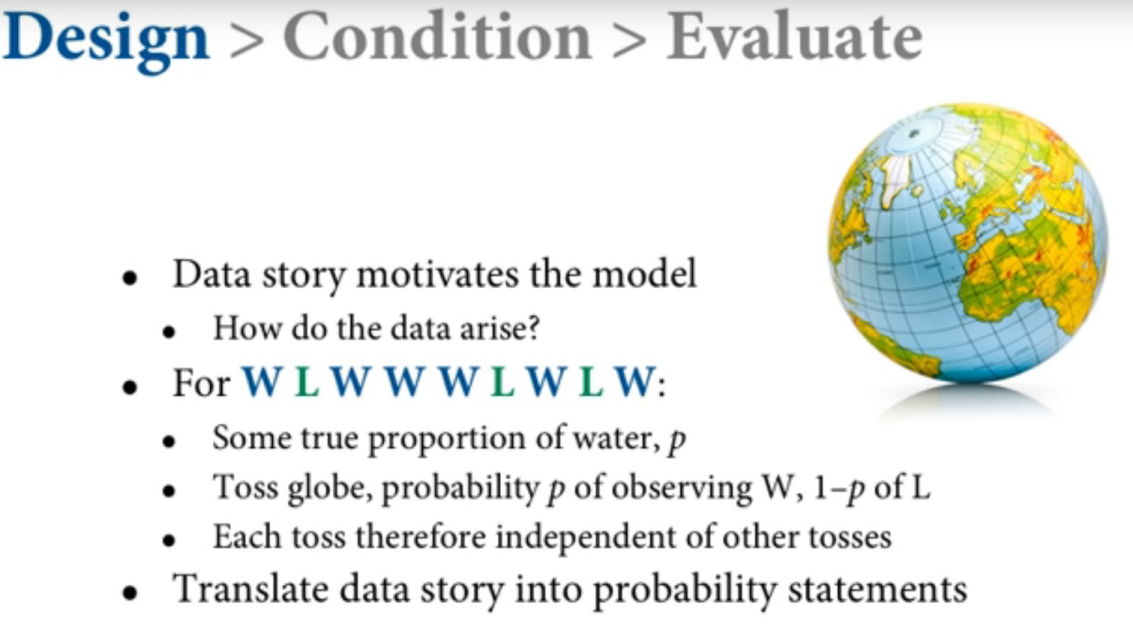In :
import numpy as np
from scipy import stats
import arviz as az
import pymc3 as pm
import matplotlib.pyplot as plt
import seaborn as sns
import pandas as pd

WARNING (theano.configdefaults): install mkl with conda install mkl-service: No module named 'mkl'
WARNING (theano.tensor.blas): Using NumPy C-API based implementation for BLAS functions.


# Glossary¶

## Data¶

In Bayesian analysis this is the fixed truth of what has been observed. There is no uncertainty or probability. In our example the globe was tossed, the sequence of events observed is Water, Land, Water Water Water, Land, Water, Land, Water

## Model¶

A model is a representation of some other thing. For example in automobile design a model is representation of "real" vehicle. Sometimes the model is life sized and made out of clay, other times it's a small toy representation made out of metal and plastic. In Bayesian analysis a model is a representation of the data generating process made of out mathematical distributions. Below is a model of the globe tossing example, written in psuedo mathematical notation.

$$proportion\_of\_water = Uniform(0,1)$$$$count\_of\_water\_observations = Binomial(proportion\_of\_water, number\_of\_tosses)$$

It is important to know that models, unlike data, are not fixed and are completely human made. Two models can exist at the same time. For example here is another model of the globe tosses

$$\lambda = Uniform(0,1)$$$$count\_of\_water\_observations = Poisson(\lambda)$$

Neither model is correct, nor is either model wrong! Both models are only a representation of the globe tossing, and justifying model choice is a core activity of Bayesian analysis.

## Bayes Theorem¶

Bayes Theorem is the probability event, taking into account past events that provide information about the event. The most generic formulation is this one.

$$P(A \mid B) = \frac{P(B \mid A) \, P(A)}{P(B)}$$

A representation that I find more intuitive however is this one $$P(parameters \mid data) = \frac{P(data \mid parameters) \, P(parameters)}{P(data)}$$

Sometimes Bayes Theorem is written as shown below. This perhaps is the most "technically correct" because it differentiates between likelihood and probability. (See likelihood and probability glossary terms below)

$$P(parameters \mid data) = \frac{\mathcal{L}(parameters \mid data) \, P(parameters)}{P(data)}$$

## Inference¶

Bayesian inference is a way to update probabilities by using Bayes formula. In other ways Inference is solving for the posterior, or left hand side, of Bayes formula. Inference can be performed numerous ways, a partial list includes

• Direct solution with point probabilities
• Conjugate prior formulas
• Grid Search
• Markov Chain Monte Carlo
• Variational Inference

Different methods of inference each have their own advantages and disadvantages. It is important to note that his also is a human choice, and different books, guides, and tutorials may show different ways of solving for the posterior.

I will be writing a guide showing how the same problem can be solved with different inference methods in the near future.

## Prior¶

The prior is the probability of an event before witnessing any data.

For our globe tossing example before tossing the globe and making any observations, how much of the globe do you think covered in water? Some people would say 70% because they took a geography class in a prior life. Others would make a reaction similar to this emoji, ¯_(ツ)_/¯, indicating they are equally unsure about all possiblities.

The choice of priors is a choice the Bayesian modeler must make. There is no fundamental truth. But luckily there is some help, for example the Stan Devs have a great tutorial providing reccomendations on choice of priors. https://github.com/stan-dev/stan/wiki/Prior-Choice-Recommendations

In :
# Create a prior in numpy
possible_proportion_of_water = np.linspace(0,1,100)
probability_of_possible = np.repeat(.1, 100)

fig,ax=plt.subplots()
ax.plot(possible_proportion_of_water, probability_of_possible)
fig.suptitle("Prior probability of Water")

Out:
Text(0.5, 0.98, 'Prior probability of Water')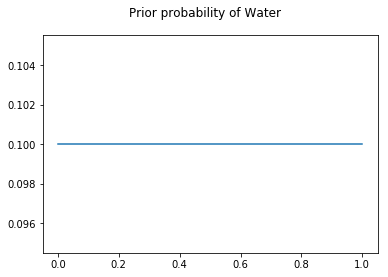## Likelihood¶

The likelihood is the probability of data, given a model and parameters. For example the likelihood given parameter of 9 Tosses, Proportion of Water of .5, and assuming a Binomal model, as shown below

In :
count_of_water_observation = 6
count_of_tosses = 9
likelihood = stats.binom.pmf(k=count_of_water_observation,
n=count_of_tosses, p=.5)
likelihood

Out:
0.16406250000000006

If plotted for all possible values for proportion of water it looks like this

In :
likelihood = stats.binom.pmf(k=count_of_water_observation,
n=count_of_tosses,
p=possible_proportion_of_water)
fig,ax=plt.subplots()
ax.plot(possible_proportion_of_water, likelihood)
fig.suptitle("Likelihood of 6 Water Observations given 9 tosses \n \
over all possible Proportions of Water")

Out:
Text(0.5, 0.98, 'Likelihood of 6 Water Observations given 9 tosses \n               over all possible Proportions of Water')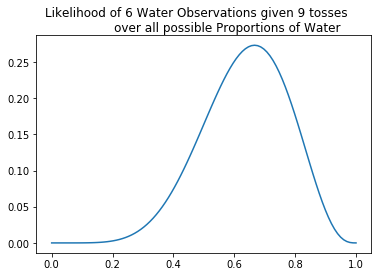Note: Likelihood is not a probability distribution, meaning that the total area of the curve is not equal to 1. This is why the syntax $\mathcal{L}(parameters \mid data)$ can be a bit more clear than $p(data \mid parameters)$

## Marginal Likelihood of Evidence/Evidence/Average Likelihood¶

The marginal likelihood of evidence is the probability of data, which is very undescriptive. In point probability problems its the probability of an event occuring without any other information, for example the probability of a postive test whether or not the patient has cancer.

An alternative formulation is as follows $$\theta = parameters \\ P(data) = \int p(Data| \theta) P(\theta)d\theta$$

In this formulation were stating "Add all the proportions of data given a parameter, scaled by their relative occurence of the parameter for all parameters"

In most inference methods however this term is readily ignored, and ends up being derived out of the inference. The reason being its non trivial to calculate analytically, and very hard to simulate in multi dimensional problems.

Due to this sometimes you see Bayes formula as written below, where the denominator is removed, and a proportional to is shown rather than an equals $$P(\theta|y) \propto P(y|\theta)P(\theta)$$

For our globe tossing example we are able to calculate the Marginal Likelihood because we are using a Grid Search Inference method can use the formulation below.

In :
# Marginal Likelihood in Grid Search formulation
numerator  = possible_proportion_of_water * likelihood
denominator = sum(numerator)
denominator

Out:
6.300000720691165

## Posterior¶

The posterior, or posterior probability, is the probability of model parameters after incorporating the data. In the our water example, we were equally sure, (or unsure) what the proportion of water on the planet was. After using our model in conjunction with the data, we the posterior distribution reflects our certainty in the parameters.

It bears repeating that the posterior distribution is a distribution of possible model parameters and not a distribution of data

In :
posterior = numerator/denominator
fig, axes =plt.subplots(1,2, figsize=(12,5))
axes.plot(possible_proportion_of_water, posterior)
axes.set_title("Posterior Probability of Proportion of Water")
axes.plot(possible_proportion_of_water, probability_of_possible)
axes.set_title("Prior Probability of Proportion of Water")

Out:
Text(0.5, 1.0, 'Prior Probability of Proportion of Water')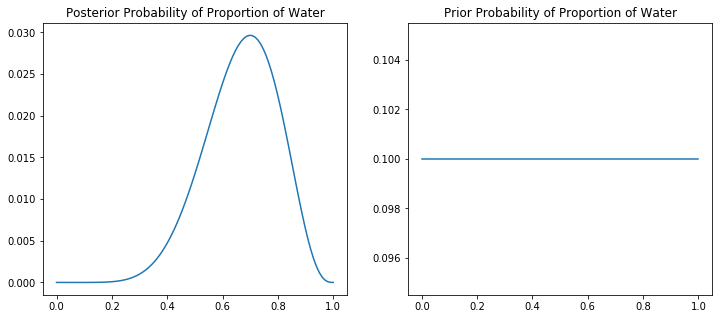## Posterior Predictive¶

An advantage of Bayesian models is being able to simulate data. The simulation of data, taking into account observed data, is called the posterior predictive. The mathmetical formulation is as follows.

$$\Pr(y' | y) = \int \Pr(y'| \theta) \Pr(\theta | y) d \theta$$

However we can simulate the posterior predictive distribution using python as well.

In :
posterior_samples = np.random.choice(possible_proportion_of_water, p=posterior, size=1000, replace=True)
posterior_predictive = stats.binom.rvs(n=9, p=posterior_samples)
counts = np.bincount(posterior_predictive)

fig, ax = plt.subplots()
ax.bar(x=np.arange(counts.shape), height=np.bincount(posterior_predictive))
ax.set_xlabel("Count of Water Observations out of 9 globe tosses")
ax.set_ylabel("Number of simulations with count")

Out:
Text(0, 0.5, 'Number of simulations with count')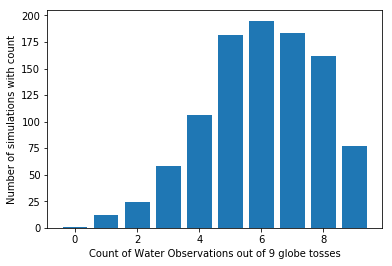It is important to note that this distribution is not probability. It is in the units of data, in this case its the distribution of "counts of water observations for 9 globe tosses". The distribution highlights the uncertainty in the globe given the 9 data points that were originally observed.

## Prior Predictive Distribution¶

Prior Predictive models are similar to posterior predictive distributions, excep that you use samples from the prior distribution of parameters, not from the posterior distribution of samples.

In mathematical notation $$p(y) = \int_{\theta} p(\theta) p(y|\theta)\text{d}\theta$$

Prior Predictive distributions are useful for checking if your model seems to be outputting reasonable data, for example in our globe tossing experiment, if we were seeing negative counts for "Number of water observations", that impossibility would suggest that the model is not appropriate for the data

In :
prior_samples = stats.uniform(0,1).rvs(1000)
prior_predictive_samples = stats.binom.rvs(n=9, p=prior_samples)
counts = np.bincount(prior_predictive_samples)

fig, ax = plt.subplots()
ax.bar(x=np.arange(counts.shape), height=np.bincount(prior_predictive_samples))
ax.set_xlabel("Count of Water Observations out of 9 globe tosses")
ax.set_ylabel("Number of simulations with count")

Out:
Text(0, 0.5, 'Number of simulations with count')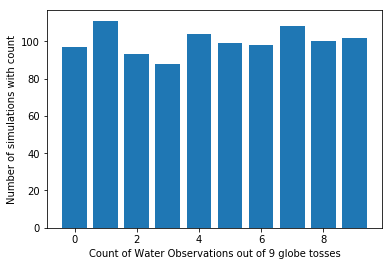## Forward Sampling¶

Forward Sampling is the same as Prior Predictive except that it includes the prior samples. So in other words while Prior Predictive is just the distribution of simulated data, Forward Sampling is that in addition to the sampled parameters from the posterior.

## Hierarchical Modeling¶

Hierarchical modeling is a mechanism in Bayesian models where you "tell" the model that data points may share similarity. The hierarchy words implies multiple "levels" of effect.

For example assume you're trying to estimate the height of an individual. Your data tells you the gender and which family the individual comes from. The relation between height and family is not constant, it's not as if coming from one family guarantees all members of a family will be double the height of another for example. But it would be unwise to assume that your samples are independent either, family genetics is well correlated with final height. But with hierarchical models allow us to split the difference, by allowing the model to "say" a families heights come from a taller distribution, than from another family with shorter family members.

The Radon Model provides a rigorous explanation accompanied by a great visual explainer

## Hierarchical Funnel¶

Hierarchical funnels are a particular parameter space topology that makes it hard for particular Inference Engines to explore the whole space. Basically what ends up happening is this, where the Bayesian sampler doesn't quite do the thing you want, but instead of your donation coin taking a long time to fall a short vertical distance, your sampler can't traverse the entire space efficiently.

Michael Betancourt wrote a full explanation on the Stan website. The tutorial has been rewritten in PyMC3 as well

# Acknowledgements¶

I would like to extend thanks to the following people for providing feedback and corrections